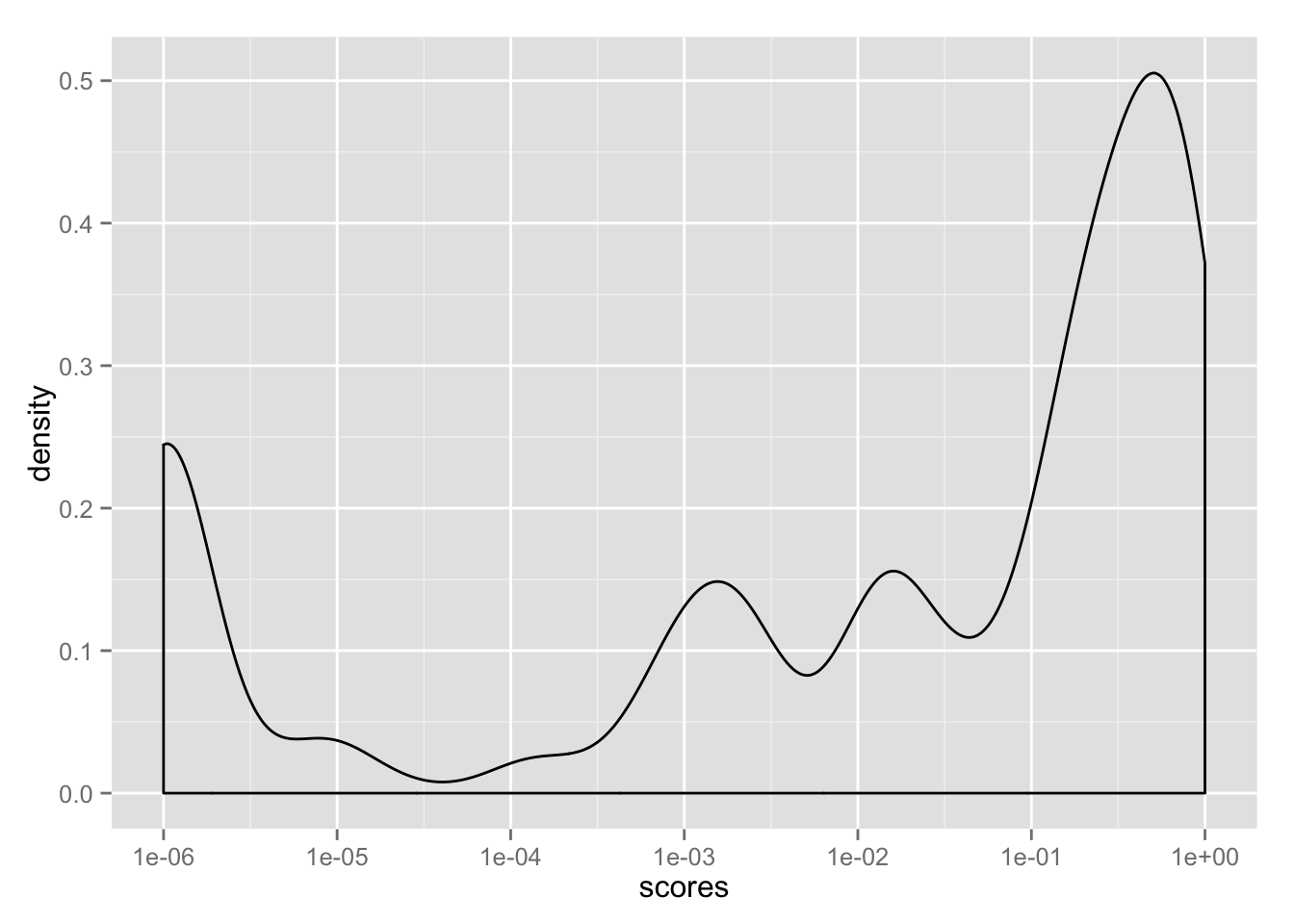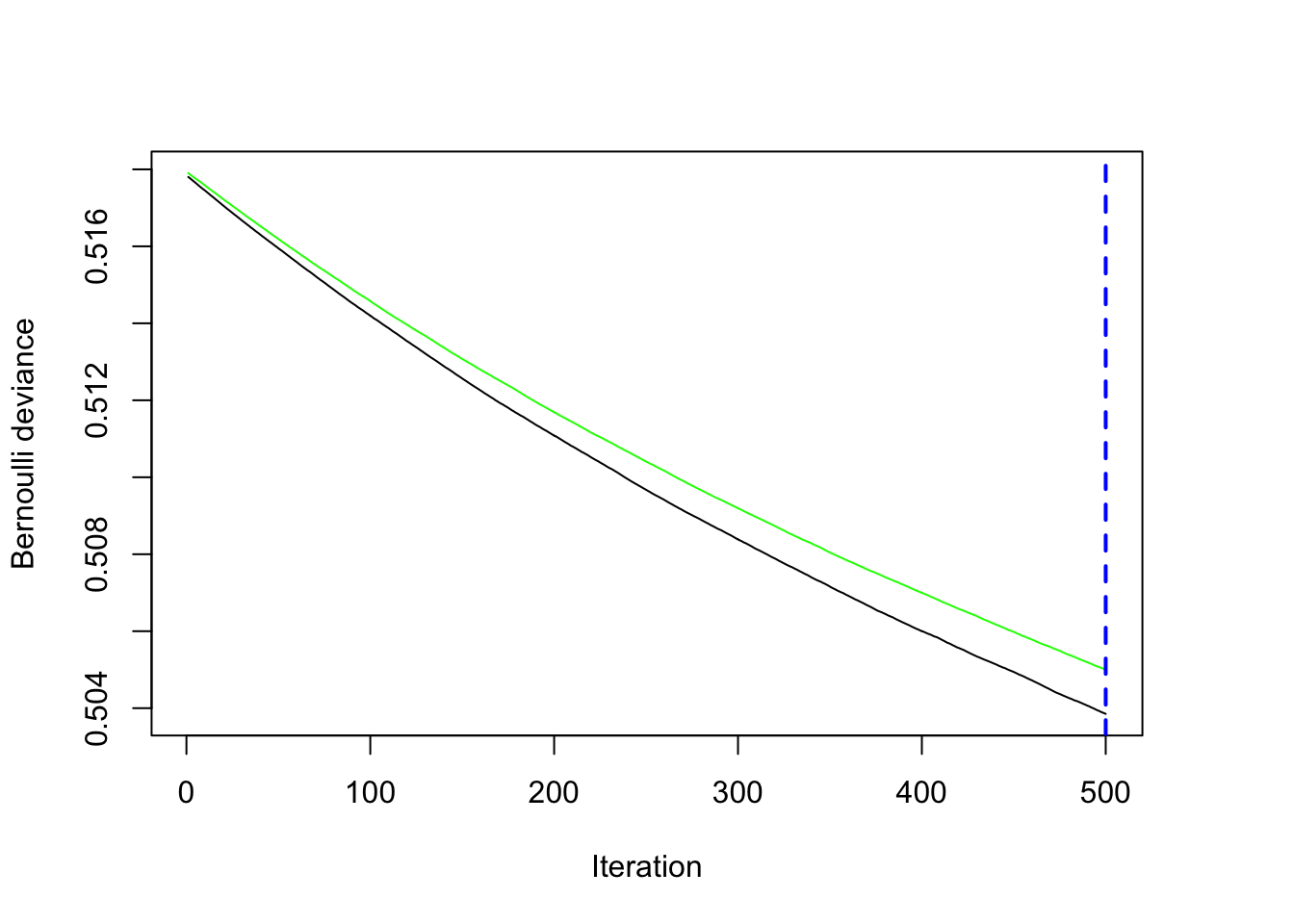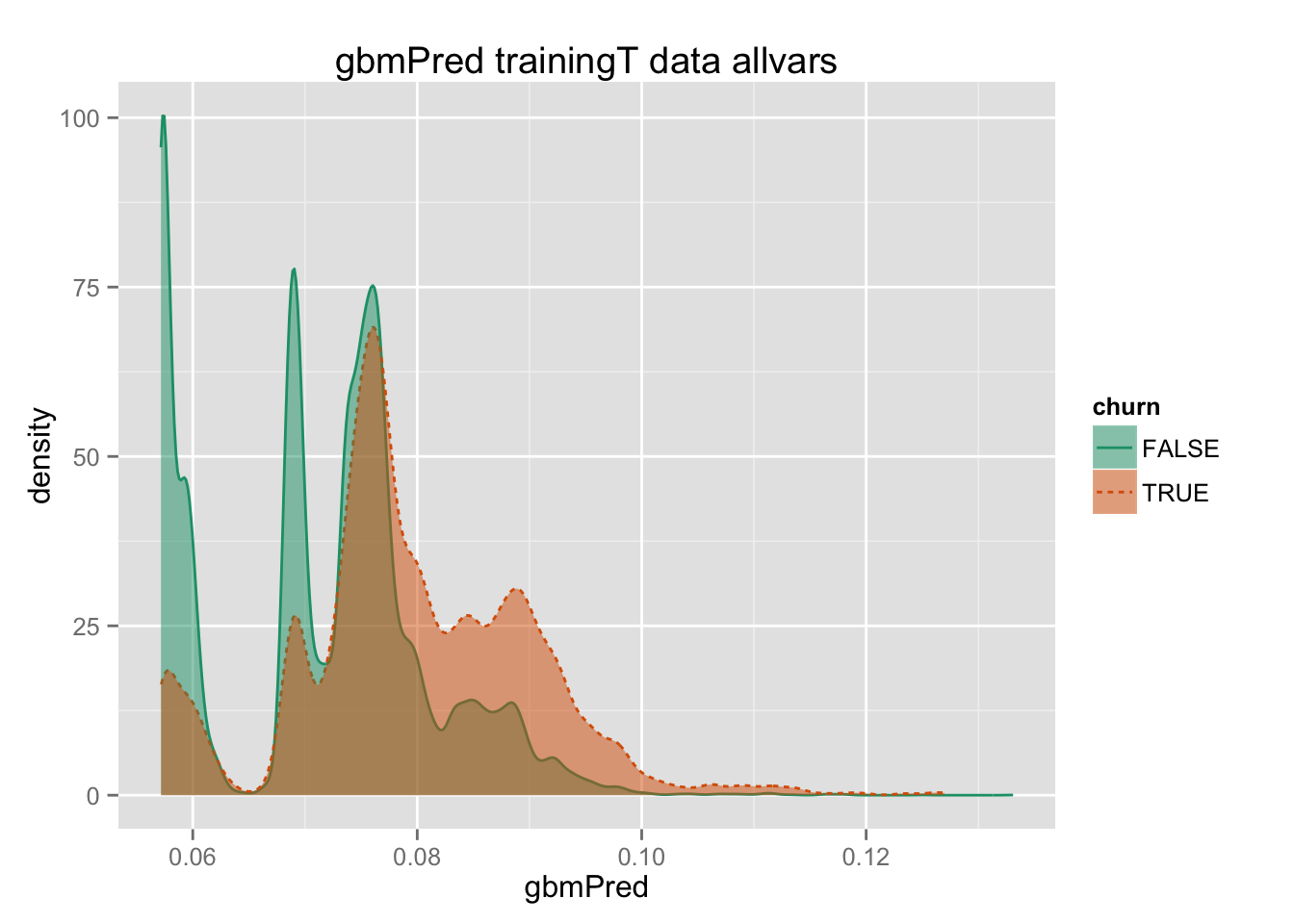``````# devtools::install_github("WinVector/vtreat")
library('vtreat')  # This library isn't public yet, intall instructions: http://www.win-vector.com/blog/2014/08/vtreat-designing-a-package-for-variable-treatment/
library('parallel')
# devtools::install_github("WinVector/WCPlots")
library('WVPlots')``````
``````## Loading required package: ggplot2
##
## Attaching package: 'gplots'
##
## The following object is masked from 'package:stats':
##
##     lowess
##

``library('gbm')``
``````## Loading required package: survival
``````# To make the html: echo "library(knitr); knit('KDD2009example.Rmd')" | R --vanilla ; pandoc KDD2009example.md -o KDD2009example.html
# Example of working with KDD2009 data (just to show library at work).
# For vtreat details see: http://www.win-vector.com/blog/2014/08/vtreat-designing-a-package-for-variable-treatment/
# and Chapter 6 of Practical Data Science with R: http://www.amazon.com/Practical-Data-Science/dp/1617291560
# For details on data see: https://github.com/WinVector/zmPDSwR/tree/master/KDD2009

# load the data as in the book
dir = '~/Documents/Projects/DataScienceBook/zmPDSwR/KDD2009/' # change this path to match your directory structure

d\$churn = churn\$V1
d\$appetency = appetency\$V1
d\$upselling = upselling\$V1
set.seed(729375)
d\$rgroup = runif(dim(d)[])

# Make the Training (modeling), Calibration (impact coding) and Test sets
dTrainM = subset(d,rgroup<=0.5)  # set for building models
dTrainC = subset(d,(rgroup>0.5) & (rgroup<=0.9)) # set for impact coding
dTest = subset(d,rgroup>0.9) # set for evaluation

# clean up
rm(list=c('d','churn','appetency','upselling','dir'))

# get variable and outcome columns
outcomes = c('churn','appetency','upselling')
vars = setdiff(colnames(dTrainM),
c(outcomes,'rgroup'))
yName = 'churn'
yTarget = 1``````

Vtreat: automatic variable treatment

``````# get the standard degrees of freedom estimates
estDF = function(d) {
vapply(d,function(v) {
df = length(unique(v)) - 1
if (is.character(v)) {
df = min(1,df)
}
df
},numeric(1))
}

# clean out the variables that are constants
dfe = estDF(dTrainM[,vars])
vars = names(dfe)[dfe>0]

# try the automatic variable treatment

set.seed(239525)

cl = parallel::makeCluster(4)

# build the data treatments on calibration data
treatments = designTreatmentsC(dTrainC,
vars,yName,yTarget,
smFactor=2.0,
parallelCluster=cl)``````
``````##  "desigining treatments Sat Aug 22 15:33:40 2015"
##  "scoring treatments Sat Aug 22 15:33:45 2015"
##  "have treatment plan Sat Aug 22 15:35:44 2015"``````
``````if(!is.null(cl)) {
parallel::stopCluster(cl)
cl = NULL
}

# prepare the training and test sets
# don't need to prepare the calibration set,
# we only used to to fit data treatment parameters
# and impact coded models
treatedTrainM = prepare(treatments,dTrainM,
pruneSig=c())
treatedTest = prepare(treatments,dTest,
pruneSig=c())
varnames = treatments\$vars

# remove the catN impact coded variables; we'll use only the catB impact coded variable
# catB: bayesian models
# catN: linear regression model against 0/1 outcome (deprecated, but still there)
isCatN = grepl("catN", varnames, fixed=TRUE)
print(paste("Number of catN variables: ", sum(isCatN)))``````
``##  "Number of catN variables:  0"``
``````varnames = varnames[!isCatN]

# yName is the y column
# convert the outcome to TRUE/FALSE
treatedTrainM[[yName]] = treatedTrainM[,yName]==yTarget
treatedTest[[yName]] = treatedTest[,yName]==yTarget``````

``````get_utility = function(model) {
model\$null.deviance - model\$deviance
}

# get the chi-squared significance of a glm model (wrt deviance)
get_significance = function(model, df=NULL) {
delta_deviance = model\$null.deviance - model\$deviance
if(is.null(df)) {
df = model\$df.null - model\$df.residual
}
pchisq(delta_deviance, df, lower.tail=FALSE)
}

# get the signal scores for the variables in a data set
# assume output is a binary variable named y
get_chiscores = function(dframe, yName, guessDF) {
nvar = length(varnames)
utils = numeric(nvar)
scores = numeric(nvar)
for(i in seq_len(nvar)) {
model = glm(paste(yName,"~",varnames[i]), dframe,

# this is a hack, added to try to adjust significance estimate for catB vars
df = 1
if((guessDF) && grepl("catB", varnames[i], fixed=TRUE)) {
df = length(unique(dframe[[ varnames[i] ]]))-1
}
utils[[i]] = get_utility(model)
scores[[i]] = get_significance(model, df)
}

sframe = data.frame(var=varnames,
scores=scores,
utilities=utils,
stringsAsFactors=FALSE)
sframe
}

#
# Plot the scores of each variable
# frm has columns var and scores
# (output of get_chiscores)
#
scoreplot = function(frm, threshold, sort=1) {
n = dim(frm)
frm\$var = reorder(frm\$var, frm\$scores*sort, FUN=sum)
frm\$goodvar = frm\$scores < threshold

ggplot(frm, aes(x=var, y=scores, ymin=0, ymax=scores, color=goodvar)) +
geom_pointrange() +
geom_hline(yintercept=threshold, color="red", linetype=2) +
scale_color_manual(values=c("TRUE"="darkgreen", "FALSE"="darkgray")) +
theme(legend.position="none")
}``````

Prepare plotting frames

``````# prepare plotting frames
treatedTrainP = treatedTrainM[, yName, drop=FALSE]
treatedTestP = treatedTest[, yName, drop=FALSE]``````

Do the variable selection, and fit gbm and glm models for comparison

``````# Use training data to score variables.
vScoresI = get_chiscores(treatedTrainM,yName,FALSE)

breaks=c(1e-6, 1e-5, 1e-4, 0.001, 0.01, 0.1, 1)
ggplot(vScoresI, aes(x=scores+1e-6)) + geom_density(adjust=0.5) + scale_x_log10("scores", breaks=breaks)````````````# threshold the significance scores
threshold = 1e-4
selVarsI = vScoresI\$var[vScoresI\$scores < threshold]
print(paste("Number of candidate variables:", nrow(vScoresI)))``````
``##  "Number of candidate variables: 448"``
``print(paste("Number of variables selected:", length(selVarsI)))``
``##  "Number of variables selected: 87"``
``print(selVarsI)``
``````##   "Var6_isBAD"
##   "Var7_clean"
##   "Var13_clean"
##  "Var65_clean"
##  "Var72_clean"
##  "Var73_clean"
##  "Var74_clean"
##  "Var81_clean"
##  "Var113_clean"
##  "Var126_clean"
##  "Var140_clean"
##  "Var144_clean"
##  "Var189_clean"
##  "Var192_catB"
##  "Var193_catB"
##  "Var199_catB"
##  "Var200_catB"
##  "Var204_catB"
##  "Var205_lev_x.sJzTlal"
##  "Var205_lev_x.VpdQ"
##  "Var205_catB"
##  "Var206_catB"
##  "Var207_lev_x.7M47J5GA0pTYIFxg5uy"
##  "Var207_lev_x.DHn_WUyBhW_whjA88g9bvA64_"
##  "Var207_lev_x.me75fM6ugJ"
##  "Var207_catB"
##  "Var210_lev_x.g5HH"
##  "Var210_lev_x.uKAI"
##  "Var210_catB"
##  "Var211_lev_x.L84s"
##  "Var211_lev_x.Mtgm"
##  "Var212_catB"
##  "Var214_catB"
##  "Var216_catB"
##  "Var217_catB"
##  "Var218_lev_x.cJvF"
##  "Var218_lev_x.UYBR"
##  "Var218_catB"
##  "Var221_lev_x.d0EEeJi"
##  "Var221_lev_x.oslk"
##  "Var221_lev_x.zCkv"
##  "Var221_catB"
##  "Var225_lev_NA"
##  "Var225_lev_x.ELof"
##  "Var225_catB"
##  "Var226_lev_x.FSa2"
##  "Var226_catB"
##  "Var227_lev_x.RAYp"
##  "Var227_lev_x.ZI9m"
##  "Var227_catB"
##  "Var228_catB"
##  "Var229_lev_NA"
##  "Var229_lev_x.am7c"
##  "Var229_lev_x.mj86"
##  "Var229_catB"``````
``````# Two cases: model with allvars, model with selected vars
strats = list('allvars'=vScoresI\$var,
'selected_vars'=selVarsI)

for(strat in names(strats)) {
selVars = strats[[strat]]
formulaS = paste(yName,paste(selVars,collapse=' + '),sep=' ~ ')
for(mname in c('gbmPred','glmPred')) {
print("*****************************")
print(date())
print(paste(mname,strat,length(selVars)))
if(mname=='gbmPred') {
start = Sys.time()
modelGBMs = gbm(as.formula(formulaS),
data=treatedTrainM,
distribution='bernoulli',
n.trees=500,
interaction.depth=2,
keep.data=FALSE,
cv.folds=5)
end = Sys.time()
diff = end-start
print(paste(strat, mname, "time to fit:", diff))
nTrees = gbm.perf(modelGBMs)
treatedTrainP[[mname]] = predict(modelGBMs,newdata=treatedTrainM,type='response',
n.trees=nTrees)
treatedTestP[[mname]] = predict(modelGBMs,newdata=treatedTest,type='response',
n.trees=nTrees)
} else {
modelglms = glm(as.formula(formulaS),
data=treatedTrainM,
)
treatedTrainP[[mname]] = predict(modelglms,newdata=treatedTrainM,type='response')
treatedTestP[[mname]] = predict(modelglms,newdata=treatedTest,type='response')
}

t1 = paste(mname,'trainingT data',strat)
print(DoubleDensityPlot(treatedTrainP, mname, yName,
title=t1))
print(ROCPlot(treatedTrainP, mname, yName,
title=t1))

t3 = paste(mname,'test data',strat)
print(DoubleDensityPlot(treatedTestP, mname, yName,
title=t3))
print(ROCPlot(treatedTestP, mname, yName,
title=t3))
print(date())
print("*****************************")
}
}``````
``````##  "*****************************"
##  "Sat Aug 22 15:36:43 2015"
##  "gbmPred allvars 448"
##  "allvars gbmPred time to fit: 7.00861508448919"
## Using cv method...``````